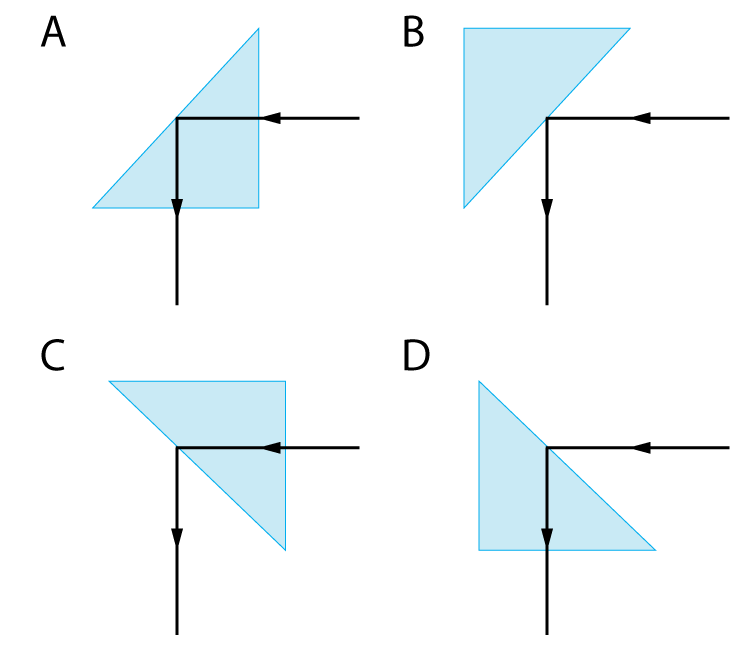µ GCSE | Refraction

10 quick questions - for GCSE and iGCSE

<

10 minutes maximum! (can you do it in 5?)

1. Which of these diagrams correctly shows the path of a ray of light through a semi-circular plastic block?2. Which of these statements best describes the path?

" As the ray enters the plastic from the air...

• A. .. it speeds up and bends away from the normal line".
• B. .. it speeds up and bends towards the normal line".
• C. .. it slows down and bends away from the normal line".
• D. .. it slows down and bends towards the normal line".

3.Which of these formulas states "Snell's Law", used to show the relationship between the angle of incidence (i) and the angle of refraction (r)?

 a) n = sin i sin r

 b) n = sin r sin i

 c) r = sin n sin i

 d) i = sin n sin r

4. What is 'n' in this equation?

• A. The density of the substance.
• B. The speed of light in the substance.
• C. The refractive index of the substance.
• D. The volume of the substance.

5. The ray of light in question 1 has an angle of incidence of 400 and an angle of refraction of 250.

What is the value of 'n'?

• A. 1.52
• B. 0.66
• C. 0.03
• D. 1.60

6. Which of the following formulas correctly states the 'critical angle' formula?

 a) n = 1 c

 b) sin c = 1 n

 c) sin n = c 1

 d) sin n = 1 c

7. Which of the following diagrams shows the path of a ray of light that has been totally internally reflected through a glass prism?8. if the glass prism has a refractive index of 1.6, what is the critical angle of the glass?

• A. 390
• B. 420
• C. 450
• D. 490

9. The prism shown in question 7 is a 450 prism, and light is totally internally reflected when it hits the surface at 450.

What is the minimum refractive index for a substance to be used in this prism?

• A. 1.0
• B. 1.4
• C. 1.5
• D. 1.6

10. Total internal reflection is used in long glass strands to carry light signals. These strands are called..

• A. Light cables.
• B. Optical fibres.
• C. Laser channels.
• D. EM wave cables.